# Consecutive Numbers

Go back to  'Numbers'

 1 Introduction to Consecutive Numbers 2 What are Consecutive Numbers? 3 Consecutive Even Numbers 4 Consecutive Odd Numbers 5 Consecutive Even and Odd Integers 6 Solved Examples on Consecutive Numbers 7 Thinking Out of the box! 8 Practice Questions on Consecutive Numbers 9 Important Notes on Consecutive Numbers 10 Frequently Asked Questions(FAQs)

We at Cuemath believe that Math is a life skill. Our Math Experts focus on the “Why” behind the “What.” Students can explore from a huge range of interactive worksheets, visuals, simulations, practice tests, and more to understand a concept in depth.

Book a FREE trial class today! and experience Cuemath’s LIVE Online Class with your child.

## Introduction to Consecutive Numbers

To understand consecutive numbers, we will first need to understand the concept of predecessors and successors.

The number that is written immediately before a number is called its predecessor.

The number that is written immediately after a number is called its successor.

## What are Consecutive Numbers?

Consecutive numbers are numbers that follow each other in order from the smallest number to the largest number.

They usually have a difference of 1 between every two numbers.

Note: The difference between any predecessor-successor pair is fixed.

Let’s look at a few examples of consecutive numbers.

Example 1: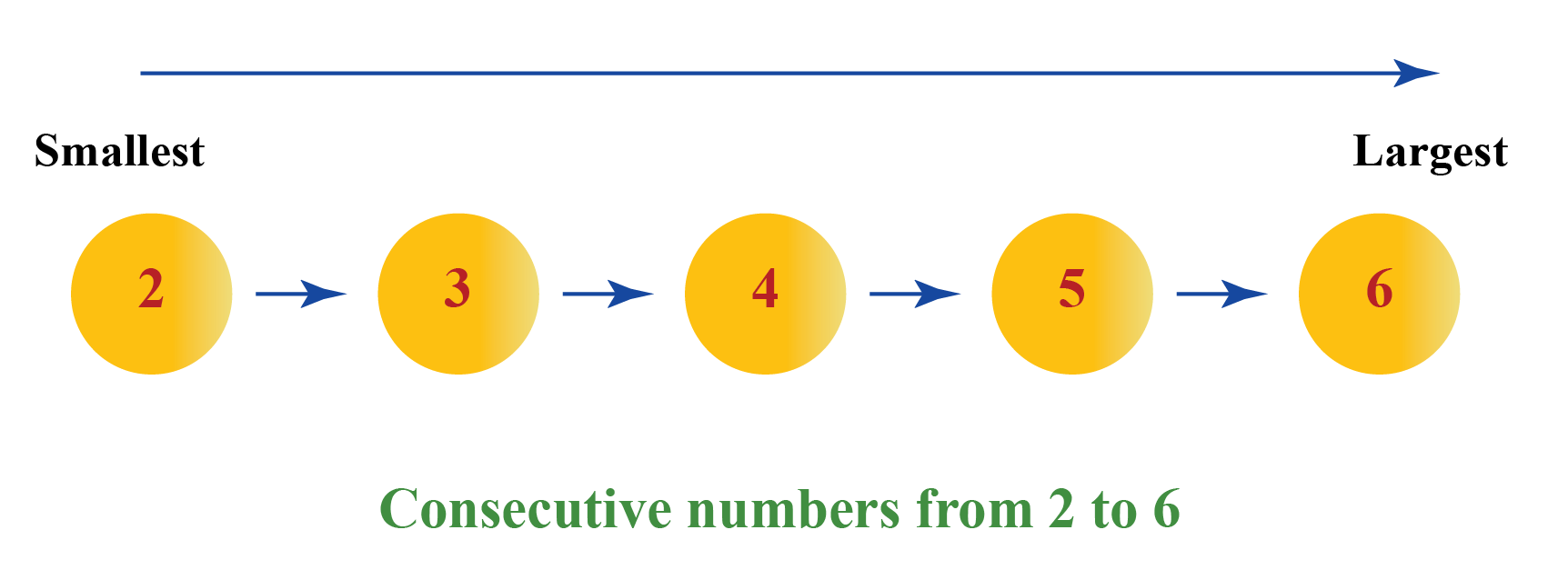In the above example, the difference between any predecessor-successor pair is 1

If we denote the 1st number as $$n$$, then the consicutive numbers in the series  will be $$n+1$$, $$n+2$$, $$n+3$$, $$n+4$$, and so on.

Here is another example for you.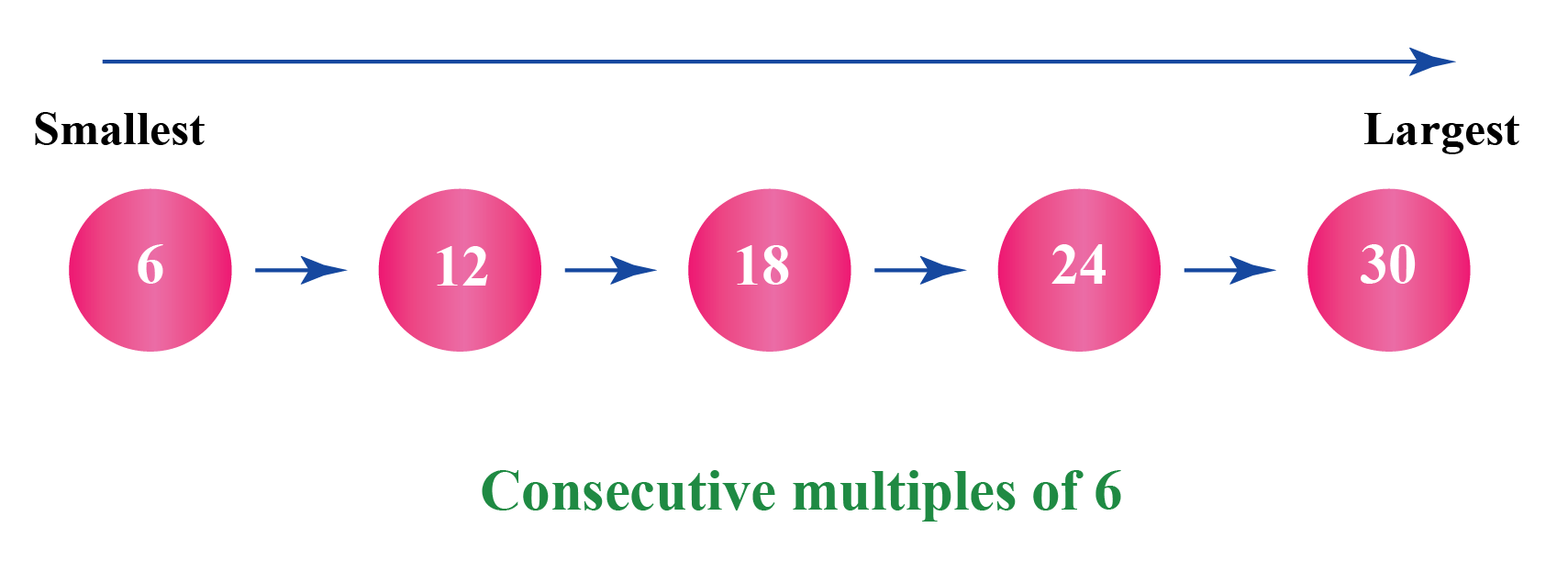The difference between any predecessor-successor pair in this example is always 6, as they are all multiples of 6

Do you think there will be an odd number in the list of consecutive multiples of 6?

## Consecutive Even Numbers

We know that even numbers  are those numbers which end in 0, 2, 4, 6, and 8

Now let us consider the set of even numbers from 2 to 12 and write them in ascending order.

The numbers are ordered as 2, 4, 6, 8, 10, 12 when written from the smallest to the largest .

We can see that the difference between any predecessor-successor pair is 2

##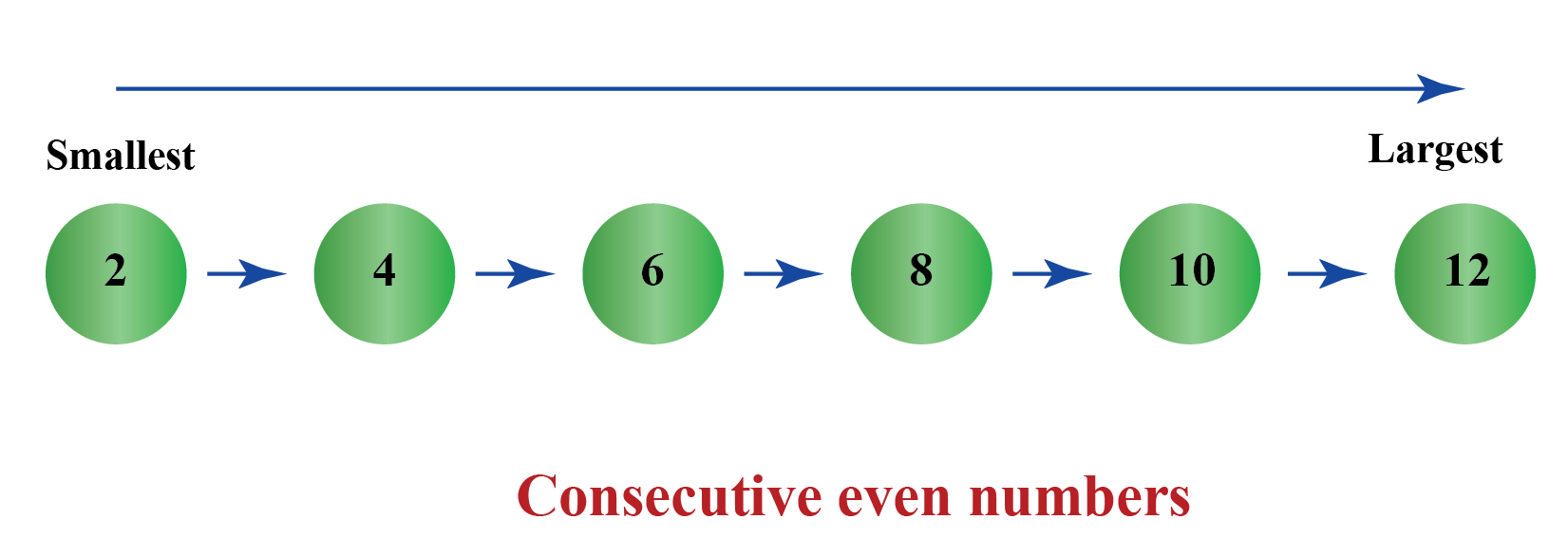Can you identify the consecutive even numbers between 20 and 30?

## Consecutive Odd Numbers

We know that odd numbers are one less or one more than the even numbers.

When we arrange them in ascending order, we can see that the difference between them is always 2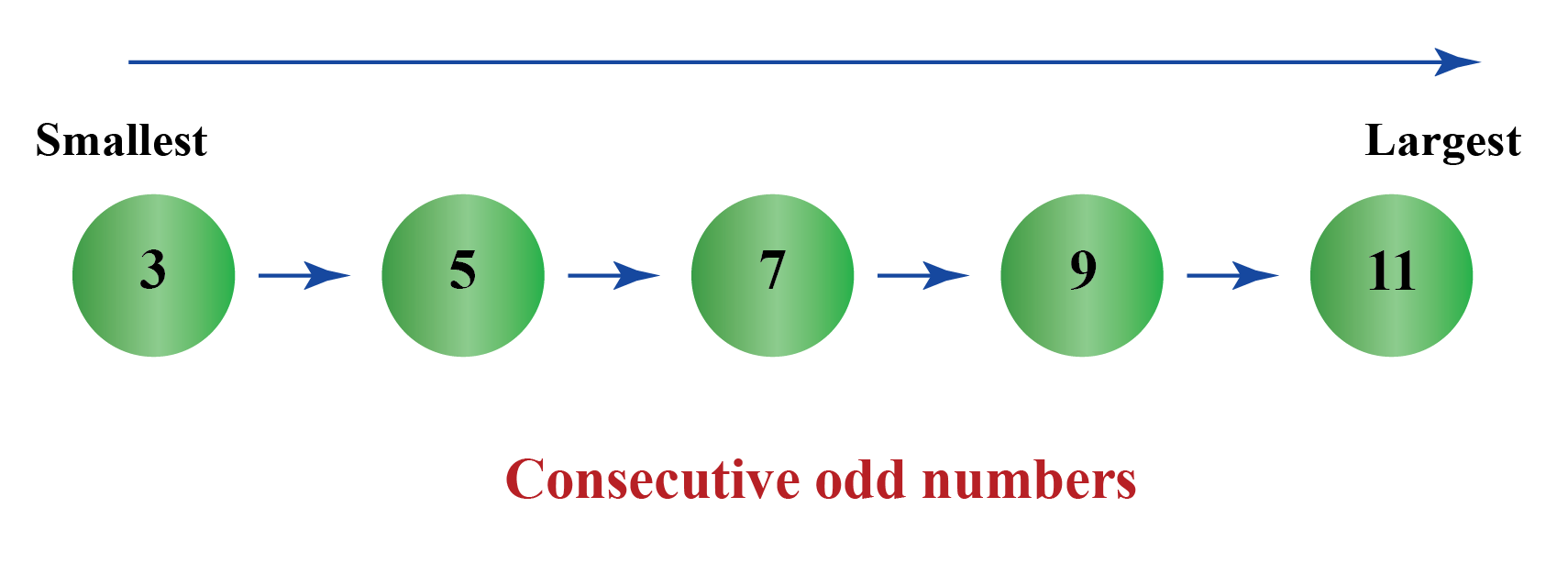In the above example, the difference between any predecessor-successor pair is 2

$$5-3 = 2$$

$$7-5 = 2$$ and so on.

## Consecutive Even and Odd Integers

We know that integers are positive and negative numbers including zero.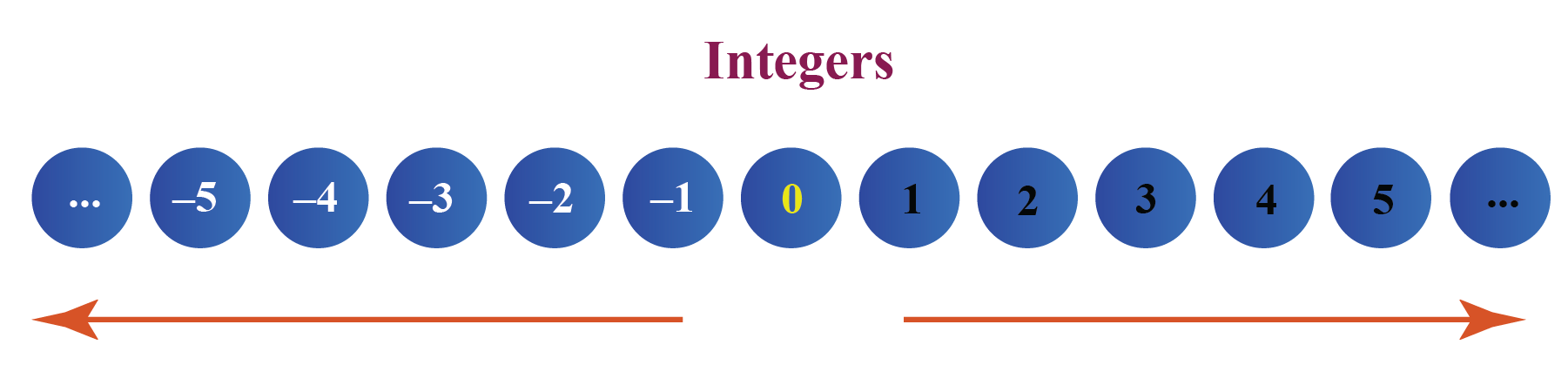### Consecutive Even Integers

We know that even integers end in $$2,4,6,8,0$$ and they include negative numbers.

Let us write the consecutive even integers between $$-6$$ and $$6$$

When we arrange these numbers in order from the least value to the greatest value, we get $$-6,-4, -2, 0, 2, 4, 6$$

Note that the difference between the successive predecessor and successor is 2

### Consecutive Odd Integers

We know that odd integers end in $$1,3,5,7,9$$ and they include negative numbers.

Let us write the consecutive odd integers between $$-5) and \(5) When we arrange these numbers in order from the least value to the greatest value, we get \( -5, -3, -1, 1, 3, 5$$

Note that the difference between the successive predecessor and successor is 2

In general, if we denote the 1st integer as $$n$$, the consecutive even or consecutive odd integers will be $$n +2$$, $$n +4$$, $$n +6$$, $$n +8$$, ...
Help your child score higher with Cuemath’s proprietary FREE Diagnostic Test. Get access to detailed reports, customised learning plans and a FREE counselling session. Attempt the test now.

## Solved Examples

 Example 1

Find the missing number in the series.

$3, 4, 5, 6, …, 8, 9, 10$

Solution:

The difference between any predecessor-successor pair in this series is 1

The predecessor of the missing number is 6

The successor of the missing number is 8

The missing number is predecessor $$+$$ difference  $$= 6 + 1 = 7$$

Alternatively, the missing number is successor $$-$$ difference $$= 8 - 1= 7$$

 $$\therefore$$ The missing number in the series is  7
 Example 2

Find the missing number in the series.

$4, 8, 12, ..., 20, 24, 28, 32$

Solution:

The difference between any predecessor-successor pair in this series is 4

The predecessor of the missing number is 12

The successor of the missing number is 20

The missing number is predecessor $$+$$ difference $$= 12 + 4= 16$$

Alternatively, the missing number is successor $$-$$ difference $$= 20 - 4= 16$$

 $$\therefore$$  The missing number in the series is 16
 Example 3

What is the third number in the given series if they are all consecutive multiples of an odd integer?

$5, 15, ___ , 25, 30$

Solution:

The difference between any predecessor-successor pair in this series is 5

The predecessor of the missing number is 15

The successor of the missing number is 25

The missing number is predecessor$$+$$ difference $$= 15 + 5= 20$$

 $$\therefore$$  The missing number is 20
 Example 4

Find the missing numbers in the following series.

$75, …, 77, 78, …, 80$

Solution:

The difference between any predecessor-successor pair in this series is 1

The predecessor of the first missing number is 75

The successor of the first missing number is 77

The missing number is predecessor$$+$$ difference $$= 75 + 1= 76$$

We see that numbers are in order: $$75, 76, 77, 78$$ and the difference is $$1$$

Thus, the next number in the series will be $$79$$

 $$\therefore$$ Missing numbers in the series are 76 and 79
 Example 5

The sum of three consecutive even numbers is 24.

What are the three numbers?

Solution:

Consecutive even numbers have a difference of 2 between them.

If the first number is $$n$$, then the second number is $$n+2$$ and the third number is $$n+4$$.

Given that their sum is  24, hence, we have:

$$n + n+2 + n+4 = 24$$

$$\implies 3n + 6 = 24$$

$$3n = 24-6 = 18$$

$$\implies n = 6$$

Therefore, the numbers are

$$n =6$$

$$n+2 = 6+2 = 8$$

$$n+4 = 6+4 = 10$$

Let us add the three numbers and verify our solution.

$$10+8+6 = 24$$

 $$\therefore$$  The numbers are 6, 8, and 10Think Tank
1. Will the sum of two consecutive numbers be odd or even?
2. Find the difference between consecutive square numbers. Do you see a pattern? What is their difference?
3. Why is the product of 3 consecutive natural numbers always divisible by 6?

CLUEless in Math? Check out how CUEMATH Teachers will explain Consecutive Numbers to your kid using interactive simulations & worksheets so they never have to memorise anything in Math again!

Explore Cuemath Live, Interactive & Personalised Online Classes to make your kid a Math Expert. Book a FREE trial class today!

## Practice Questions

Here are a few activities for you to practice.

More Important Topics
Numbers
Algebra
Geometry
Measurement
Money
Data
Trigonometry
Calculus
More Important Topics
Numbers
Algebra
Geometry
Measurement
Money
Data
Trigonometry
Calculus
Learn from the best math teachers and top your exams

• Live one on one classroom and doubt clearing
• Practice worksheets in and after class for conceptual clarity
• Personalized curriculum to keep up with school

0